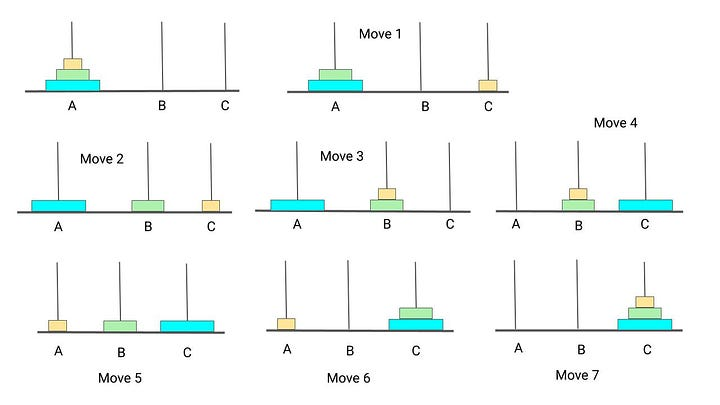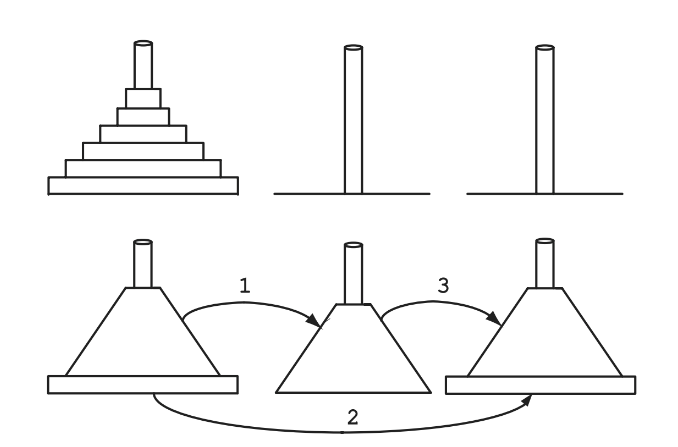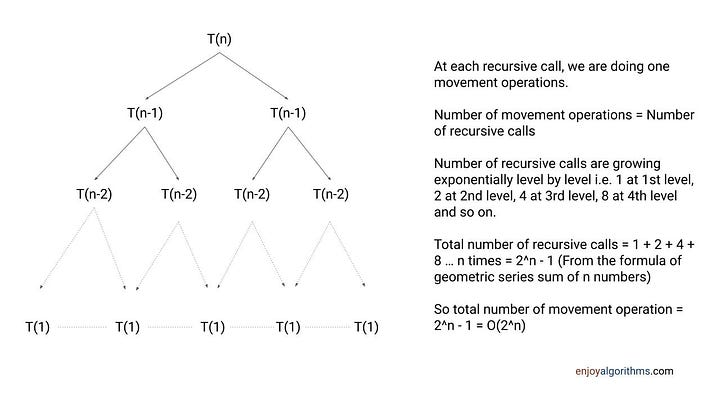# Tower of Hanoi Puzzle

The Tower of Hanoi puzzle is attributed to the French mathematician Edouard Lucas, who came up with it in 1883. His formulation involved three pegs and eight distinctly-sized disks stacked on one of the pegs from the biggest on the bottom to the smallest on the top.

### Problem Description

Given a stack of n disks arranged from largest on the bottom to smallest on top placed on a rod A, together with two empty rods B and C. The objective is to move the n disks from rod A to rod C using rod B.

• Only one disk can be moved at a time.
• No disk can be placed on top of a smaller disk. In other words, moves are allowed only if we place smaller disks on top of larger disks.
• Each move consists of taking the top disk from one of the rods and placing it on top of another rod i.e. a disk can only be moved if it is the topmost disk on a rod.

Example: The solution for 3 disks takes 7 steps:#### Solution idea and steps

If we observe, to move the bottom disk, we need to move all the disks above it to another rod first. Then we can move the bottom disk to the remaining empty rod and move the n − 1 smaller disks back on top of it. In other words, one way to move the stack of rod A to rod C is:

• Move top n-1 disks from rod A to rod B.
• Move the bottom disk from rod A to rod C.
• Move n-1 disks from rod B to rod C.In the above procedure, we are solving the problem using the solution of smaller sub-problems i.e. using recursion. How? Let’s think! Initial problem was to move n disks from rod A to rod C. Now it gets reduced to moving 2 smaller subproblems of size n - 1:

• Subproblem 1: Moving top n-1 disks from rod A to rod B using C
• Moving bottom disk from rod A to rod C
• Subproblem 2: Moving n-1 disks from rod B to rod C using A

Suppose we use the method towerHanoi(n, A, C, B) to move n rings from rod A to C using rod B. The base case is a situation when we have more disks to move i.e. n = 0. Think!

#### Solution Pseudocode

``````void towerHanoi(int n, String A, String C, String B)
{
if(n > 0)
{
towerHanoi(n - 1, A, B, C)
move(n, A, C)
towerHanoi(n - 1, B, C, A)
}
}
``````

So first recursive call moves n - 1 disks from A to B using C. So after that call n - 1 disks are in rod B in order of size and rod A contains the nth disk i.e, the largest one. So, now move the nth disk from A to C using method move(n, A, C). Then again by the 2nd recursive call move n-1 disk from B to C using A. So, after this, C contains all disks in order of size.

#### Time complexity analysis

Let the time complexity for moving n disks is T(n). There are 2 recursive calls for n-1 disks and one move operation to move a disk from A to C. If n = 1, we simply move the single disk directly.

For n > 1, T(n) = 2 T(n — 1) + 1, where T(0) = 0 and T(1) = 1

We can solve this equation using the substitution method. Here are the steps:

``````=> T(n) = 2 * [2 * T(n - 2) + 1] + 1 = 4 * T(n - 2) + 3
=> T(n) = 4 * [2 * T(n - 3) + 1] + 3c = 8 * T(n - 3) + 7
... and so on ....
=> T(n) = 2^i * T(n - i) + (2^i - 1)
When i = n, It will reach the base case i.e. T(0).
=> T(n) = 2^n * T(0) + (2^n- 1)
=> T(n) = 2^n * 0 + (2^n - 1)
=> T(n) = 2^n - 1
=> T(n) = O(2^n)
``````

So it has exponential time complexity which is computationally very expensive! For a single increase in problem size, the time required is double the previous one. This is not due to the fact that this particular algorithm is poor; in fact, it is not difficult to prove that this is the most efficient algorithm possible for this problem.

Analysis of tower of Hanoi using recursion tree method:An interesting story: In the original version of the Tower of Hanoi puzzle, as published by its inventor, the French mathematician Édouard Lucas, in the 1880s, the world will end after 64 disks have been moved by monks from a mystical Tower of Brahma. Assuming that the monks do not eat, sleep, or die and move one disk per minute, the world will end after about 3 ·10^13 years, which is more than one thousand times longer than the estimated age of the universe.

#### Space complexity analysis

The total space used is dominated by the space for the recursion stack. Let c denote the space used by a copy of the local variables. Since the space used by the first call is released when it returns, the recurrence relation for the space used: S(n) = c (If n =1), S(n) = S(n −1) + c (If n > 1).

The solution to this recurrence relation is O(n). From another analogy, the space used by the call stack is equal to the depth of the recursion tree, which is equal to n. So space complexity = n*c = O(n).

### Critical ideas to think about!

• Can we implement the above recursive solution using iteration? Do we need to use a stack data structure for the iterative implementation?
• Can we think to solve the tower of Hanoi problem using a bitwise algorithm? Here is a hint: Total number of moves for n disks is equal to 2^n — 1, where all bit values are set to 1. So disk positions can be determined more directly from the binary representation of the move number i.e. the initial state start with all digits 0, and the final state end with all digits 1.
• Explore different variations of this puzzle based on several restrictions.
• What are the various applications of the tower of Hanoi in problem-solving?

Enjoy learning, Enjoy algorithms!

Share on social media:

© 2020 Code Algorithms Pvt. Ltd.